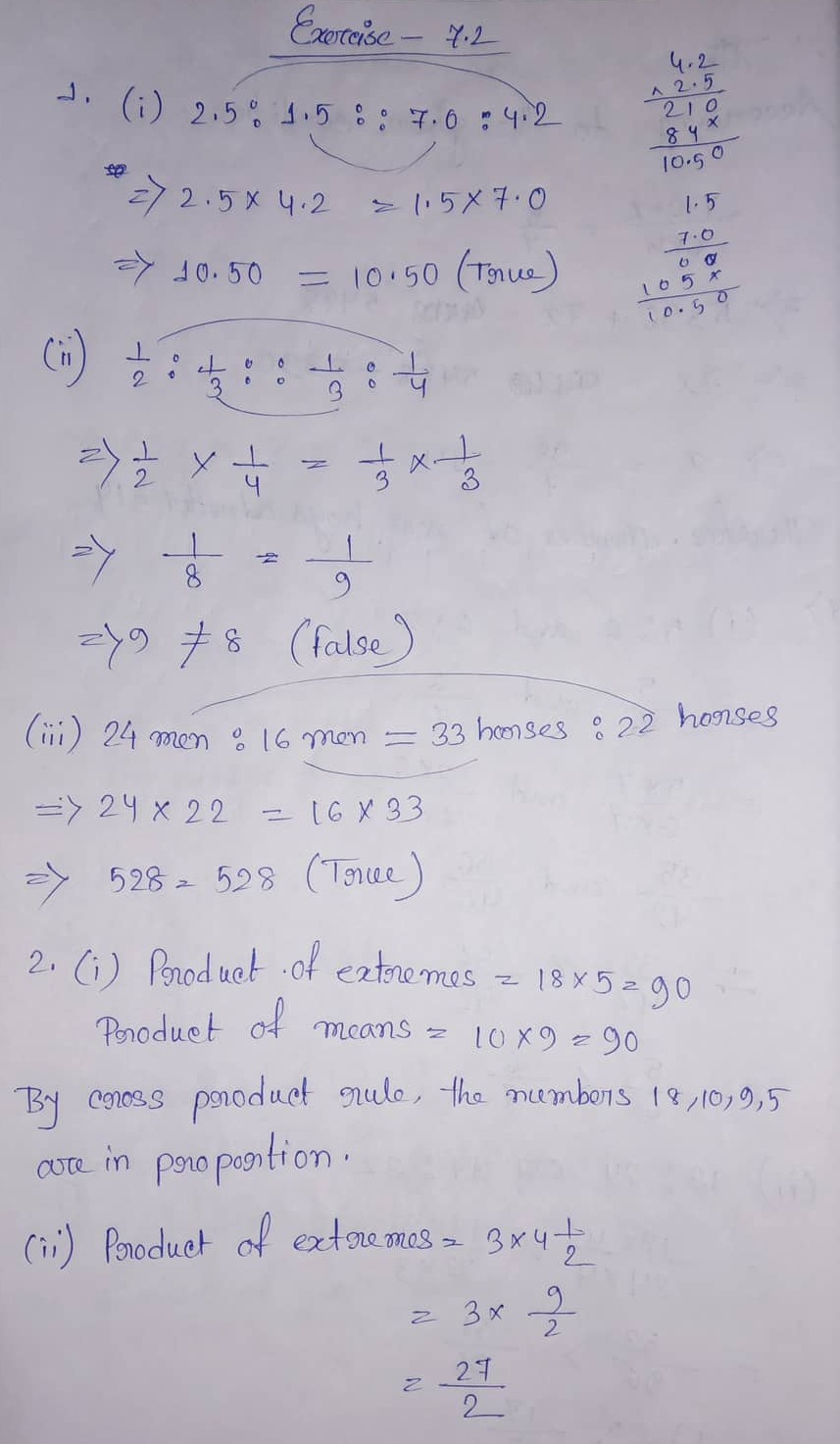# ML Aggarwal CBSE Solutions Class 7 Math Seventh Chapter Ratio and Proportion Exercise 7.2

### ML Aggarwal CBSE Solutions Class 7 Math 7th Chapter Ratio and Proportion Exercise 7.2

(1) Which of the following statements are true?
(i) 2.5 : 1.5 :: 7.0 : 4:2
(ii) 1/2 : 1:3 = 1/3: 1/4
(iii) 24 men : 16 men = 33 horses : 22 horses.

(2) Check whether the following numbers are in proportion or not:
(i) 18,10,9,5
(ii) 3, 7/2, 4, 9/2
(iii) 0.1,0.2, 0.3, 0.6

(3) Find x in the folllowing proportions:
(i) x:4 = 9:12
(ii) 1/13 : x :: 1/2 : 1/5
(iii) 3.6:0.4 = x:0.5

(4) Find the fourth proportional to
(i) 42, 12, 7
(ii) 1/3, 1/4, 1/5
(iii) 3 kg, 12 kg, 15 kg

SOLUTION Number 1, 2, 3 & 4:(5) Check whether 7, 49, 343 are in continued proportion or not.

(6) Find the third proportional to
(i) 36, 18
(ii) 21/4, 7
(iii) 3.2, 0.8

(7) The ratio between the length and width of a rectangular sheet of paper is 7:5. If the width of the sheet is 20.5 cm, find the length.

(8) The ages of Amit and Archana are in the ratio 4:5. If Amit is 4 years 8 months old, find the age of Archana.

SOLUTION Number 5, 6, 7 & 8:Updated: January 10, 2020 — 2:52 pm